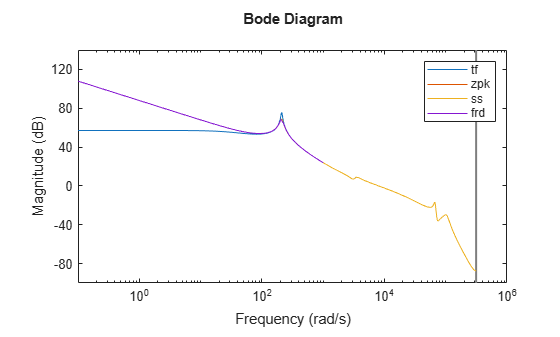Documentation

## Recommended Model Type for Building Block Diagrams

This example shows how choice of model type can affect numerical accuracy when interconnecting models.

You can represent block diagram components with any model type. However, certain connection operations yield better numerical accuracy for models in `ss` form.

For example, interconnect two models in series using different model types to see how different representations introduce numerical inaccuracies.

Load the models `Pd` and `Cd`. These models are ninth-order and second-order discrete-time transfer functions, respectively.

`load numdemo Pd Cd`

Compute the open-loop transfer function `L = Pd*Cd` using the `tf`, `zpk`, `ss`, and `frd` representations.

```Ltf = Pd*Cd; Lzp = zpk(Pd)*Cd; Lss = ss(Pd)*Cd; w = logspace(-1,3,100); Lfrd = frd(Pd,w)*Cd;```

Plot the magnitude of the frequency response to compare the four representations.

```bodemag(Ltf,Lzp,Lss,Lfrd) legend('tf','zpk','ss','frd')```The `tf` representation has lost low-frequency dynamics that other representations preserve.